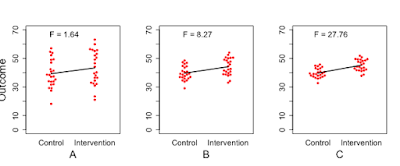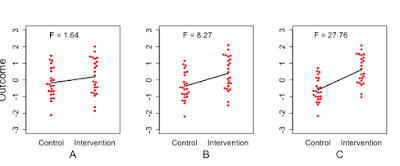## Friday, 24 November 2017

### Intuitive explanations of statistical concepts for novices #2

In my last post, I gave a brief explainer of what the term 'Analysis of variance' actually means – essentially you are comparing how much variation in a measure is associated with a group effect and how much with within-group variation.

The use of t-tests and ANOVA by psychologists is something of a historical artefact. These methods have been taught to generations of researchers in their basic statistics training, and they do the business for many basic experimental designs. Many statisticians, however, prefer variants of regression analysis. The point of this post is to explain that, if you are just comparing two groups, all three methods – ANOVA, t-test and linear regression – are equivalent. None of this is new but it is often confusing to beginners.

Anyone learning basic statistics probably started out with the t-test. This is a simple way of comparing the means of two groups, and, just like ANOVA, it looks at how big that mean difference is relative to the variation within the groups. You can't conclude anything by knowing that group A has a mean score of 40 and group B has a mean score of 44. You need to know how much overlap there is in the scores of people in the two groups, and that is related to how variable they are. If scores in group A range from to 38 to 42 and those in group B range from 43 to 45 we have a massive difference with no overlap between groups – and we don't really need to do any statistics! But if group A ranges from 20 to 60 and group B ranges from 25 to 65, then a 2-point difference in means is not going to excite us. The t-test gives a statistic that reflects how big the mean difference is relative to the within-group variation.  What many people don't realise is that the t-test is computationally equivalent to the ANOVA. If you square the value of t from a t-test, you get the F-ratio*.Figure 1: Simulated data from experiments A, B, and C.  Mean differences for two intervention groups are the same in all three experiments, but within-group variance differs

Now let's look at regression. Consider Figure 1. This is similar to the figure from my last post, showing three experiments with similar mean differences between groups, but very different within-group variance. These could be, for instance, scores out of 80 on a vocabulary test. Regression analysis focuses on the slope of the line between the two means, shown in black, which is referred to as b. If you've learned about regression, you'll probably have been taught about it in the context of two continuous variables, X and Y, where the slope b, tells you how much change there is in Y for every unit change in X. But if we have just two groups, b is equivalent to the difference in means.

So, how can it be that regression is equivalent to ANOVA, if the slopes are the same for A, B and C? The answer is that, just as illustrated above, we can't interpret b unless we know about the variation within each group. Typically, when you run a regression analysis, the output includes a t-value that is derived by dividing b by a measure known as the standard error, which is an index of the variation within groups.

An alternative way to show how it works is to transform data from the three experiments to be on the same scale, in a way that takes into account the within-group variation. We achieve this by transforming the data into z-scores. All three experiments now have the same overall mean (0) and standard deviation (1). Figure 2 shows the transformed data – and you see that after the data have been rescaled in this way, the y-axis now ranges from -3 to +3, and the slope is considerably larger for Experiment C than Experiment A. The slope for z- transformed data is known as beta, or the standardized regression coefficient.Figure 2: Same data as from Figure 1, converted to z-scores

The goal of this blogpost is to give an intuitive understanding of the relationship between ANOVA, t-tests and regression, so I am avoiding algebra as far as possible. The key point is when you are comparing two groups, t and F are different ways of representing the ratio between variation between groups and variation within groups, and t can be converted into F by simply squaring the value. You can derive t from linear regression by dividing the b or beta by its standard error - and this is automatically done by most stats programmes. If you are nerdy enough to want to use algebra to transform beta into F, or to see how Figures 1 and 2 were created, see the script Rftest_with_t_and_b.r here.

How do you choose which statistics to do? For a simple two-group comparison it really doesn't matter and you may prefer to use the method that is most likely to be familiar to your readers. The t-test has the advantage of being well-known – and most stats packages also allow you to make an adjustment to the t-value which is useful if the variances in your two groups are different. The main advantage of ANOVA is that it works when you have more than two groups. Regression is even more flexible, and can be extended in numerous ways, which is why it is often preferred.

Further explanations can be found here:

*It might not be exactly the same if your software does an adjustment for unequal variances between groups, but it should be close. It is identical if no correction is done.

1.Link to R script seems broken.

1.Thx for letting me know. I think it's now fixed. Fingers crossed...

2.Seems fine now.

2.This comment has been removed by the author.

3.I've had some helpful comments on Twitter that provide useful supplementary material. Isabella Ghement and others raised questions about what to do when variances of the two groups are unequal, noting Welch's t-test and Welch's ANOVA can handle unequal variances in the two groups. For linear regression, one can fit a model which uses different error variances, sigma1 and sigma2 for the two groups. This is a nice summary http://www.ghement.ca/Handout.pdf. Also Jonas Lindelov on stackexchange: https://stats.stackexchange.com/questions/303269/common-statistical-tests-as-linear-models. Apologies if I have omitted others - Twitter is too transient a medium, which is why I am copying here!

1.And yet more here:
http://www.sbirc.ed.ac.uk/cyril/glm/GLM_lectures.html
Thanks Cyril!